###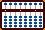SQUARE ROOTS

This tutorial dates back to October 2003 when I wrote it as a chapter for Dave Bernazzani's Soroban/Abacus Handbook. I recreate it here since Dave's pages have been offline as of May, 2006. - T.P.H

When it comes to extracting square roots, it's true; I can't remember the last time I needed to extract the square root of a large number and apply it to anything practical. I guess I just wanted to learn the technique because I think it's so amazing that it can be done on the abacus. In fact not only can it be done, using an abacus makes it easy.

There are different techniques that can be used to extract square roots using an abacus. I'll use the one that Takashi Kojima seems to favor in his book, Advanced Abacus - Theory and Practice. Rather than get muddled up with a bunch of algebraic formulas, I'll stick to how it's done on the abacus. For me this makes it more interesting. It certainly makes it less confusing because I don't understand algebraic formulas anyway.

In order to illustrate the technique, I'll use the terminology square number, root number and square. The square number refers to the number from which the square root is to be extracted. It's always set on the right-hand side of the abacus. The root number refers to the number that eventually forms our answer. It's always set on the left-hand side of the abacus. To square (or squaring) a number refers to multiplying a number by itself as in the example, 3 x 3 = 9.

In preparing to find the square root of a number, whether it be on paper or on the abacus, the number is normally separated into pairs of digits. In the example  17,362.236  starting at the decimal point and moving left and right the numbers would be separated  1'  73'  62'. 23'  60. Because of the constraints of using a small cyber abacus, we will do the separation and pairing mentally. Kojima does the same in his book.

Although the technique involves only simple mathematics and is therefore quite easy to do, the extraction process is fairly involved and labor intensive. For this reason trying to explain how it all works may be an exercise in confusion. It might just be better to jump ahead and learn from the examples below. However, by way of a brief explanation:

• Begin by placing the number from which the square root is to be extracted onto the right hand side of the abacus. This number on the right is the "square number".

• Group the square number into pairs as described above.

• Look at the first group of paired numbers (or number) and determine the largest perfect square that is less than or equal to the group. Subtract the perfect square from the group.

• Dealing again with the perfect square, take its square root and place it on the left hand side of the abacus. This number on the left is the "root number" and will eventually form our answer.

• Double the root number and divide it into the next group in the square number on the right hand side. Place the quotient along-side the first root number.

• Mentally square the quotient and subtract it from the square number on the right hand side.

• Continue the process until the square number on the right is used up. In the event the square number is not a perfect square, when the decimal is reached further groups of 00 may be added and the process continued.

• When finished go back and halve the numbers that you doubled in the root number on the left. What remains will be the square root answer.

Confused? I think I would be. But take heart. Doing the following examples below on your abacus should clarify things.

### Example 1.  \/ 1156 = ?

Step 1: Set 1156 on the right hand side of your abacus. This is the square number. If there's room, pair it off 11   56 or do it mentally. Because there are two groups (11 & 56) the answer will have two whole numbers possibly followed by a decimal in the answer. (Fig.1)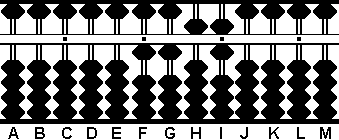Fig.1 ```Step 1 A B C D E F G H I J K L M . . . .   0 0 0 0 0 1 1 5 6 0 0 0 0 ```

Step 2: Now look at the first group of numbers. (In this case the 11 on rods F & G.) Find the largest perfect square that is less than or equal to 11. The answer is 9. Subtract 9 from 11 leaving 2. Set the square root of 9 (namely 3) somewhere on the left-hand side of the abacus, in this case on rod B. This forms the first part of the root number. (Fig.2)Fig. 2 ```Step 2 A B C D E F G H I J K L M . . . .   0 3 0 0 0 1 1 5 6 0 0 0 0 - 9   0 3 0 0 0 0 2 5 6 0 0 0 0 ```

Step 3: Double the 3 on rod B to equal 6 and divide 6 into 25 on rods G & H.  6 goes into 25 four times with a remainder. Place 4 on rod C. Multiply 6 x 4 to equal 24 and subtract 24 from 25. That leaves 16 on rods H & I. (Fig.2)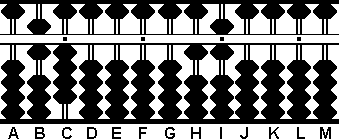Fig. 3 ```Step 3 A B C D E F G H I J K L M . . . .   0 6 0 0 0 0 2 5 6 0 0 0 0 4 -2 4   0 6 4 0 0 0 0 1 6 0 0 0 0```

Step 4: Now mentally square the 4 on rod C and subtract the product from the remainder on the right.  4 x 4 = 16. Perfect. Subtract 16 from rods H & I. (Fig.4)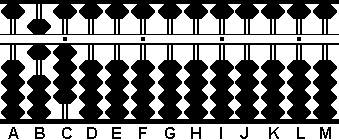Fig.4 ```Step 4 A B C D E F G H I J K L M . . . .   0 6 4 0 0 0 0 1 6 0 0 0 0 (4) - 1 6   0 6 4 0 0 0 0 0 0 0 0 0 0 ```

Step 5: Almost done. In Step 3 we doubled the 3 on rod B to equal 6. Now it must be halved to its original 3 and we have the answer to the problem on rods B & C. (Fig.5)

 The answer 34 on rods B & C.Fig. 5 ``` ____ \/ 1156 = 34 ```

* I've included this next example because it illustrates where it's possible to get a little confused and take a wrong turn. While the problem is easily overcome, it is something to watch for.

### Example 2.  \/ 74,529 = ?

Step 1: Set 74,529 on the right hand side of your abacus. This is the square number. If there's room, pair it off  7   45   29  or do it mentally.   **Notice the odd pairing. The number 7 stands alone and will be considered the first group.**   Because there are three groups  (7, 45 & 29)  we know the answer will have three whole numbers possibly followed by a decimal.  (Fig.6)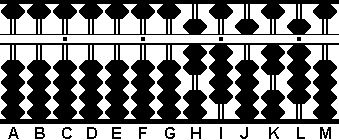Fig. 6 ```Step 1 A B C D E F G H I J K L M . . . .   0 0 0 0 0 0 0 7 4 5 2 9 0 ```

Step 2: Look at the first group of numbers. (In this case there's only the 7 on rod H.) Find the largest perfect square that is less than or equal to 7. The answer is 4. Subtract 4 from 7 leaving 3. Set the square root of 4 (namely 2) somewhere on the left-hand side of the abacus, in this case on rod D. This forms the first part of the root number. (Fig.7)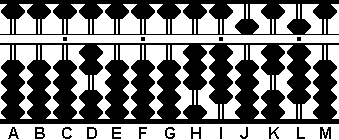Fig. 7 ```Step 2 A B C D E F G H I J K L M . . . .   0 0 0 2 0 0 0 7 4 5 2 9 0 - 4   0 0 0 2 0 0 0 3 4 5 2 9 0 ```

Step 3: Now double the 2 on rod D to equal 4 and divide 4 into 34 on rods G & H. Convention has it that  34 ÷ 4 = 8  with a remainder. But....

 * This is where it gets interesting. The technique requires that after dividing  4 into 34  you have to subtract the square of the quotient. In other words, you have to have enough left over in the remainder. See it? If you choose  34 ÷ 4 = 8  there will only be 25 remaining on rods I & J. That's not enough left over to subtract  8 x 8 = 64.  However, if you choose  34 ÷ 4 = 7  there will be 65 remaining on rods I & J. And 65 leaves you enough left over to subtract the square  7 x 7 = 49.  See what I mean? Even though it's the correct answer to the division problem, you can't use 8 because you can't subtract its square. You have to go with 7. While this kind of problem doesn't always come up, it's something that can happen.

Okay, 7 it is. 4 goes into 34 seven times with a remainder. Place 7 on rod E. Multiply 4 x 7 to equal 28 and subtract 28 from 34 on rods H & I. With 65 left over on I & J there's enough to work with in Step 4. (Fig.8)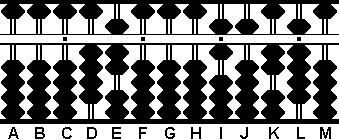Fig. 8 ```Step 3 A B C D E F G H I J K L M . . . .   0 0 0 4 0 0 0 3 4 5 2 9 0 7 - 2 8   0 0 0 4 7 0 0 0 6 5 2 9 0 ```

Step 4: Now that we've ensured there's enough left over to work with, take the 7 on rod E and subtract its square from the remainder on the right.  7 x 7 = 49. Subtract 49 from 65 on rods I & J leaving 16. (Fig.9)Fig. 9 ```Step 4 A B C D E F G H I J K L M . . . .   0 0 0 4 7 0 0 0 6 5 2 9 0 (7) - 4 9   0 0 0 4 7 0 0 0 1 6 2 9 0 ```

Step 5: Next, double the 7 on rod E to equal 14. Place 14 on rods E & F. (Fig.10)Fig.10 ```Step 5 A B C D E F G H I J K L M . . . .   0 0 0 4 7 0 0 0 1 6 2 9 0 + 7   0 0 0 4 1 4 0 0 1 6 2 9 0```

Step 6: Now divide 414 on rods D, E & F into 1629, on the right. It looks like 414 goes into 1629  three times with a remainder. Place 3 on rod G and multiply it by the 4 on rod D to equal 12. Subtract 12 from 16 on rods I & J. leaving 4.

Step 6(a): Now multiply 3 by the 1 on rod E to equal 3 and subtract 3 from rod J.

Step 6(b): Next multiply 3 by the 4 on rod F to equal 12 and subtract 12 from rods J & K. (Fig.11)

 The result after steps 6, 6(a) and 6(b)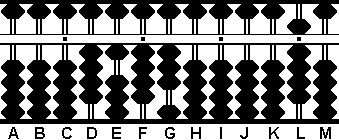Fig.11 ```Steps 6, 6(a), 6(b) A B C D E F G H I J K L M . . . .   0 0 0 4 1 4 0 0 1 6 2 9 0 3 - 1 2   0 0 0 4 1 4 3 0 0 4 2 9 0 - 3   0 0 0 4 1 4 3 0 0 1 2 9 0 -1 2   0 0 0 4 1 4 3 0 0 0 0 9 0 ```

Step 7: The rest is easy. All that's left in this step is to mentally square the 3 and subtract the product from the remainder on the right. 3 x 3 = 9. Subtract 9 from rod L. (Fig.12)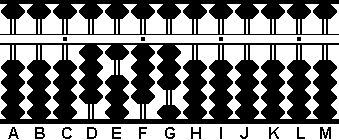Fig.12 ```Step 7 A B C D E F G H I J K L M . . . .   0 0 0 4 1 4 3 0 0 0 0 9 0 (3) - 9   0 0 0 4 1 4 3 0 0 0 0 0 0 ```

Step 8: Nearly done. In steps 3 and 5 we doubled portions of the root number. Now they must be halved to their original numbers. Take half of 4 on rod D to equal 2. Take half of 14 on rods E & F to equal 7 and place it on rod E. Move the 3 on G to rod F. And there's the answer. (Fig.13)

 The final answer 273 on rods D, E & F.Fig.13 ``` _____ \/ 74529 = 273 ```

### Example 3.  \/ 39.3129 = ?

Step 1: This example involves a decimal. Set 39.3129 on the right hand side of your abacus. This is the square number. If there's room, pair it off  39. 31 29  or do it mentally. The first group is the whole number 39 on rods H & I. From this we know the answer will have one whole number. Anything that follows will be a decimal. (Fig.14)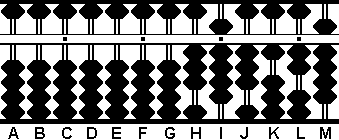Fig.14 ```Step 1 A B C D E F G H I J K L M . . . .   0 0 0 0 0 0 0 3 9 3 1 2 9 ```

Step 2: Now look at the first group of numbers. (In this case the 39 on rods H & I.) Find the largest perfect square that is less than or equal to 39. The answer is 36. Subtract 36 from 39 leaving 3. Set the square root of 36 (namely 6) somewhere on the left-hand side of the abacus, in this case on rod C. This forms the first part of the root number. (Fig.15)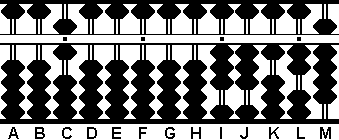Fig.15 ```Step 2 A B C D E F G H I J K L M . . . .   0 0 6 0 0 0 0 3 9 3 1 2 9 - 3 6   0 0 6 0 0 0 0 0 3 3 1 2 9 ```

Step 3: Double the 6 on rod C to equal 12 and place 12 on rods B & C. (Fig.16)Fig.16 ```Step 3 A B C D E F G H I J K L M . . . .   0 0 6 0 0 0 0 0 3 3 1 2 9 + 6   0 1 2 0 0 0 0 0 3 3 1 2 9 ```

Step 4: Divide 12 into 33 on rods I & J. 12 goes into 33 twice with a remainder. Place 2 on rod D. Multiply 12 x 2 to equal 24 and subtract 24 from 33. That leaves 9 on rod J. (Fig.17)Fig.17 ```Step 4 A B C D E F G H I J K L M . . . .   0 1 2 0 0 0 0 0 3 3 1 2 9 2 - 2 4   0 1 2 2 0 0 0 0 0 9 1 2 9 ```

Step 5: Mentally square the 2 on rod D and subtract the product from the remainder on the right. 2 x 2 = 4. Subtract 4 from rod K. (Fig.18)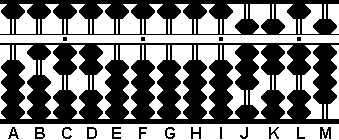Fig.18 ```Step 5 A B C D E F G H I J K L M . . . .   0 1 2 2 0 0 0 0 0 9 1 2 9 (2) - 4   0 1 2 2 0 0 0 0 0 8 7 2 9 ```

Step 6: Double the 2 on rod D to equal 4 leaving 124 on rods B, C & D. (Fig.19)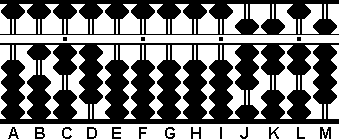Fig.19 ```Step 6 A B C D E F G H I J K L M . . . .   0 1 2 2 0 0 0 0 0 9 1 2 9 + 2   0 1 2 4 0 0 0 0 0 8 7 2 9 ```

Step 7: Now divide 124 on rods B, C & D into 8729 on the right. It looks like 124 goes into 8729 seven times with a remainder. Place 7 on rod E and multiply it by the 1 on rod B to equal 7. Subtract 7 from 8 on rod J leaving 1.

Step 7(a): Multiply 7 by 2 on rod C to equal 14. Subtract 14 from rods J & K.

Step 7(b): Multiply 7 by 4 on rod D to equal 28. Subtract 28 from rods K & L.  (Fig.20)

 The result after steps 7, 7(a) and 7(b)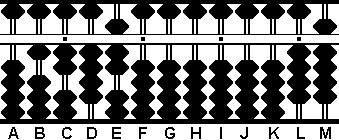Fig.20 ```Steps 7, 7(a), 7(b) A B C D E F G H I J K L M . . . .   0 1 2 4 0 0 0 0 0 8 7 2 9 7 - 7   0 1 2 4 7 0 0 0 0 1 7 2 9 - 1 4   0 1 2 4 7 0 0 0 0 0 3 2 9 - 2 8   0 1 2 4 7 0 0 0 0 0 0 4 9 ```

Step 8: Almost there. Mentally square the 7 on rod E and subtract the product from the remainder on the right. 7 x 7 = 49. Subtract 49 from rods L & M.  (Fig.21)Fig.21 ```Step 8 A B C D E F G H I J K L M . . . .   0 1 2 4 7 0 0 0 0 0 0 4 9 (7) - 4 9 0 1 2 4 7 0 0 0 0 0 0 0 0 ```

Step 9: The last step. In steps 3 and 6 we doubled portions of the root number. Now they must be halved to their original numbers. Take half of 12 on rods B & C to equal 6. Place 6 on rod C. Take half of 4 on rod D to equal 2. And there's the answer.  (Fig.22)

 The answer 6.27 on rods C, D & E.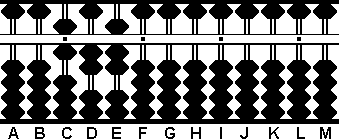Fig.22 ``` _______ \/ 39.3129 = 6.27 ```